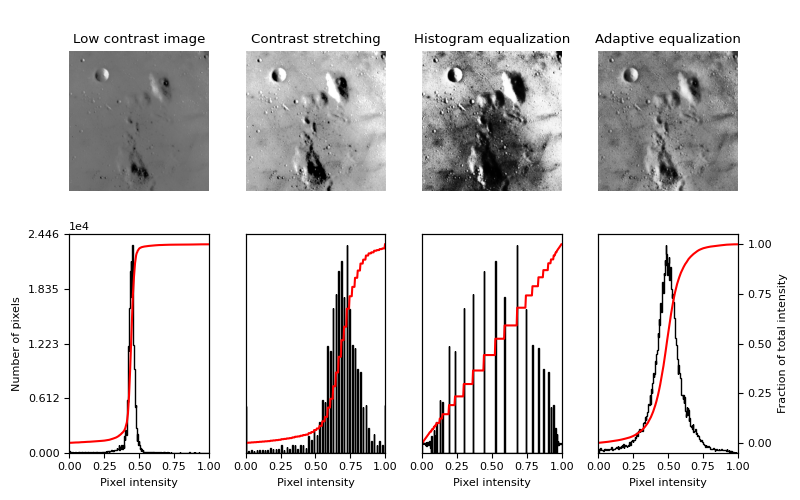# Histogram Equalization#

This examples enhances an image with low contrast, using a method called histogram equalization, which “spreads out the most frequent intensity values” in an image . The equalized image has a roughly linear cumulative distribution function.

While histogram equalization has the advantage that it requires no parameters, it sometimes yields unnatural looking images. An alternative method is contrast stretching, where the image is rescaled to include all intensities that fall within the 2nd and 98th percentiles .```import matplotlib
import matplotlib.pyplot as plt
import numpy as np

from skimage import data, img_as_float
from skimage import exposure

matplotlib.rcParams['font.size'] = 8

def plot_img_and_hist(image, axes, bins=256):
"""Plot an image along with its histogram and cumulative histogram.

"""
image = img_as_float(image)
ax_img, ax_hist = axes
ax_cdf = ax_hist.twinx()

# Display image
ax_img.imshow(image, cmap=plt.cm.gray)
ax_img.set_axis_off()

# Display histogram
ax_hist.hist(image.ravel(), bins=bins, histtype='step', color='black')
ax_hist.ticklabel_format(axis='y', style='scientific', scilimits=(0, 0))
ax_hist.set_xlabel('Pixel intensity')
ax_hist.set_xlim(0, 1)
ax_hist.set_yticks([])

# Display cumulative distribution
img_cdf, bins = exposure.cumulative_distribution(image, bins)
ax_cdf.plot(bins, img_cdf, 'r')
ax_cdf.set_yticks([])

return ax_img, ax_hist, ax_cdf

img = data.moon()

# Contrast stretching
p2, p98 = np.percentile(img, (2, 98))
img_rescale = exposure.rescale_intensity(img, in_range=(p2, p98))

# Equalization
img_eq = exposure.equalize_hist(img)

# Display results
fig = plt.figure(figsize=(8, 5))
axes = np.zeros((2, 4), dtype=object)
axes[0, 0] = fig.add_subplot(2, 4, 1)
for i in range(1, 4):
axes[0, i] = fig.add_subplot(2, 4, 1+i, sharex=axes[0,0], sharey=axes[0,0])
for i in range(0, 4):
axes[1, i] = fig.add_subplot(2, 4, 5+i)

ax_img, ax_hist, ax_cdf = plot_img_and_hist(img, axes[:, 0])
ax_img.set_title('Low contrast image')

y_min, y_max = ax_hist.get_ylim()
ax_hist.set_ylabel('Number of pixels')
ax_hist.set_yticks(np.linspace(0, y_max, 5))

ax_img, ax_hist, ax_cdf = plot_img_and_hist(img_rescale, axes[:, 1])
ax_img.set_title('Contrast stretching')

ax_img, ax_hist, ax_cdf = plot_img_and_hist(img_eq, axes[:, 2])
ax_img.set_title('Histogram equalization')

ax_img, ax_hist, ax_cdf = plot_img_and_hist(img_adapteq, axes[:, 3])